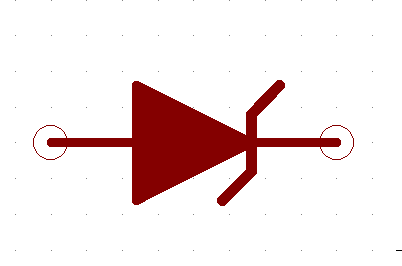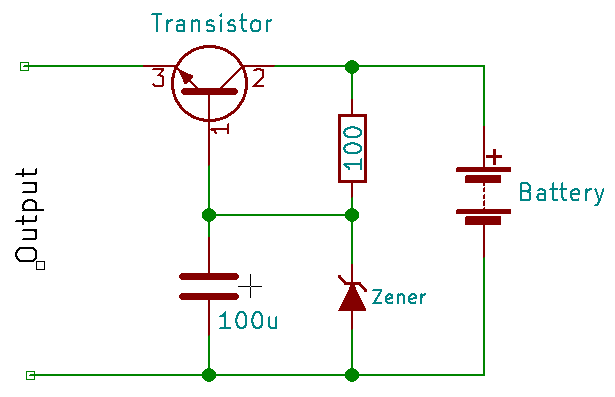## Regulating Voltage in PSLab

Electronic components are highly sensitive to voltages and currents across them. Most of the devices in the current market work in the voltage levels of 3.3V, 5V, 12V and 15V. If they are provided with a different voltage than the one required by the vendor, they would not function. If the voltage supplied is higher, they might burn off. The PSLab device requires separate voltage levels such as 3.3V and 5V for its operation.

There are commercial voltage regulators available in the market designed with advanced feedback techniques and models. But we can create out own voltage regulator. In this blog post, I am going to introduce you to a few basic models capable of regulating voltage to a desired level.

Current implementation of PSLab device uses a voltage regulator derived using a zener-resistor combination. This type of regulators have a higher sensitivity to current and their operation may vary when the supplied or the drawn current is lower than the expected values. In order to have a stable voltage regulation, this combination needs to be replaced with a much stable transistor-zener combination.

Before go into much details, let’s get to know a few basic concepts and devices related to.

Zener DiodeZener diode is a type of diode which has a different operational behavior than the general diode. General diodes allow current to flow only in one direction. If a current in the reverse is applied, they will break and become unusable after a certain voltage level known as Breakdown Voltage. But Zener diodes are specifically designed to function desirably once this break down voltage has been passed and unlike general diode, it can recover back to normal when the voltage is removed or reduced.

TransistorThis is the game changing invention of the 20th century. There are two types of Bipolar Junction Transistors (BJT) available in the market. They are known as NPN and PNP transistors. The difference is based on the polarity of diodes used.

An NPN transistor can be modeled as a combination of two diodes –[NP → PN]– and a PNP transistor can be modeled as –[PN → NP]– using two diodes.

There are three pins to take notice in BJTs. They are illustrated in the diagram shown here;

1. Base
2. Collector
3. Emitter

The amazing fact about BJTs is that the amount of current provided to the Base terminal will control the flow of current going through Collector and Emitter. Also note that always there is a voltage drop across the Base terminal and the Emitter terminal. This typically takes a value of 0.7 V

Voltage Divider

This is the most basic type of voltage regulator. It simply divides the voltage supplied by the battery with the ratio R1:R2. In the following configuration, the output voltage can be calculated using the voltage division rule;Which is equal to 12 * 100/(100+200) = 4 VThere is a huge drawback with this design. The above calculation is valid only if there is no load impedance is present at the output terminals.

Generally there will be a load impedance and the supplied voltage cannot be easily calculated as the load impedance is unknown to the regulator.

Resistor-Zener Voltage Regulator

Due to the load dependability of the previous model with the load, an improved model can be introduced as follows. This is the current implementation of voltage regulator in PSLab device.Unlike the previous model, this model ensures that the output voltage will be maintained constant across the output terminals within a range of supply voltage values.

Let’s assume the supply voltage is increased. Then the current flow through the zener diode will increase in order to maintain a constant voltage across the output terminals. In case if the supply voltage drops, then the zener current will decrease and a stable voltage across the output terminals will be maintained.

This design also comes with a slight draw back. This can be explained using the characteristic curve of a zener diode. (Figure is taken from : http://www.electronics-tutorials. ws/diode/diode_7.html)For a zener diode to maintain a constant voltage level across output terminals, there should be a minimum current flowing through the diode. If this current is not flowing in the zener, there won’t be a regulation. Assume there is a very low load impedance. Then the current supplied by the source will find an easier path to flow other than through the diode. This will affect the regulatory circuit and the desired voltage will not appear across the output terminals.

To compensate the drawback, a much improved design is available using transistors.

Transistor-Zener Voltage RegulatorThis is the proposed improvement to the voltage regulatory circuit in PSLab device. In this model, the zener diode is taken away from the load circuit as the current to the load is supplied from the transistor directly. This avoids current limitations to the zener diode had in the previous model and transistor acts as a bridge.

A small current through the Base terminal (1) will support a higher current flow through the output terminal via Collector (2) and Emitter (3). This amplification ratio is in the range of few hundreds for a typical BJT.

A capacitor has been added to compensate ripples from the supply source. If a higher current flow is required through the output terminals, the NPN transistor can be replaced by a Darlington pair.

Using a 5.6 V zener diode and MMBT3904/6 transistors, this model has been implemented in the newest version of PSLab device. They will be supplying a constant voltage of +/- 5V to V+ and V- pins in the device.